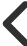Ideas Post### Hcarstens

@hcarstens# [geometries] of ideas

using geometries to create ideas

read down the list, when you find yourself pausing, when you find yourself wondering what-if, that's your idea!

## 1. Point

types of points
moving points
number line of points
routes along points

## 2. Vertical Line

line through the columns of a table
line connecting points
line avoiding points
distance between points
moving average

## 3. Horizontal Line

line through the rows of a table (notice how rows and columns contain different things and so lines through them mean different things: within a column and across rows)
line connecting points
line avoiding points
distance between points
moving average

## 4. Circular

Sin, Cosine
Fast Fourier Transform (decomposition)
Area

## 5. Rectangular

Area
Contained within
Kept out
What happens if doubles?
What happens if halves?

## 6. Bayesian

Bayes theorem (current evidence updates prior probabilities) can be thought of as a geometry - so fun! Thank you 3 blue 1 brown...

## 7. Time

Add a time dimension to any of the above geometries to create motion
Point in time
Horizontal velocity
Vertical velocity
Vector
Momentum

## 8. Third Dimension

Add a z axis to create 3 dimensional geometries like cubes, cylinders and cones
Add a force to z axis to create classical mechanics

## 9. Transformations

Add a transformation to any geometry or motion to come up with a derivative ideaPreview
0 Like.0 CommentHashamand 2 more liked this015932700